# Chapter 5 – Algebra, equations, functions and graphs

Consider the following statements and click to reveal the answer.

1. Simplify the following expressions: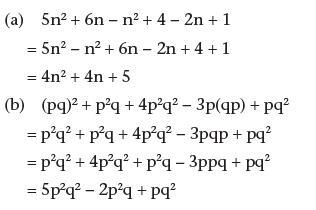2. Find the general term for each of these sequences: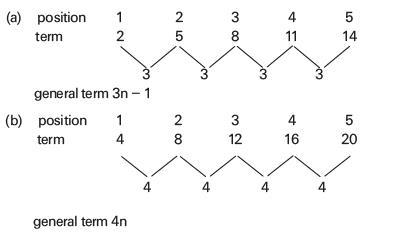3. Solve the following pairs of simultaneous equations: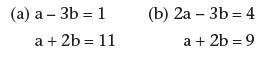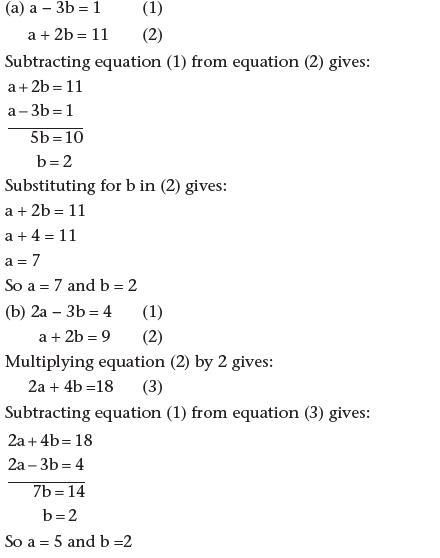4. Find the gradients and the y-intercepts of the graphs of the following equations: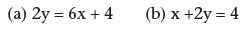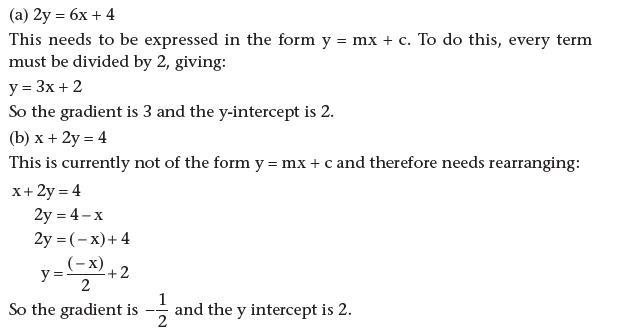5. Find the general term for the following sequence: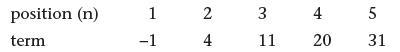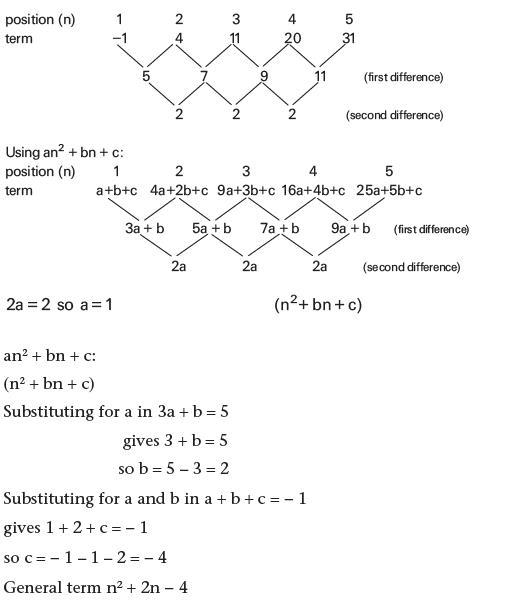6. What is a conjecture?

A conjecture is a hypothesis: something that has been surmised or deduced.

7. What different methods can be used to solve simultaneous linear equations?